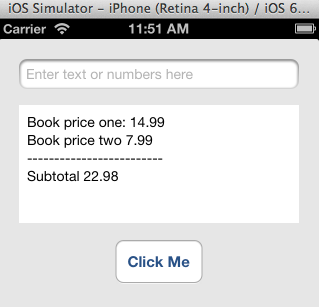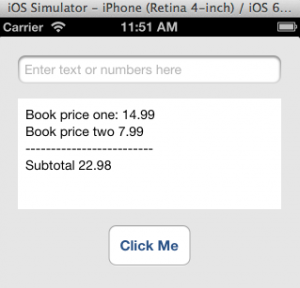# The NSDecimalNumber ClassThe NSDecimalNumber Class is a subclass of the NSNumber class and has methods for performing arithmetic operations without loss of precision and with predictable rounding behavior. This makes it a better choice for representing currency than C’s floating-point data type like double. NSDecimalNumber objects are immutable, which means you cannot change their value after they’ve been created.

 To practice source code presented in this lesson, you should download and unzip this file.Launch the project (CodePractice.xcodeproj) and enter code in the viewController.m file’s buttonTapped method, then click the “Click Me” button to execute the method’s code.

### The decimalNumberWithMantissa: Method

This method creates and returns an NSDecimalNumber object and you must provide three arguments for it to work.

##### Syntax

Argument Purpose
mantissa The number to be converted to a new decimal object.
exponent The number of decimal places for the new decimal object.
isNegative A Boolean value (YES/NO) specifying whether the sign of the number is negative.

Here is an example of how to create and initialize an NSDecimalNumber object using the decimalNumberWithMantissa: method, then display it in the UITextField control.

Code Output
```NSDecimalNumber *bookPrice = [NSDecimalNumber decimalNumberWithMantissa:1495
exponent:-2
isNegative:NO];

// Convert the NSDecimalNumber to a string object before assigning it to the UITextField control
self.inputBoxOne.text = [bookPrice stringValue];
```
14.95

### The decimalNumberWithString: Method

This method creates and returns an NSDecimal object and you must provide a numeric string argument for it to work.

Code Output
```NSDecimalNumber *bookPrice;
bookPrice = [NSDecimalNumber decimalNumberWithString:@"208.32"];

// Convert the NSDecimalNumber to a string object before assigning it to the UITextField control
self.inputBoxOne.text = [bookPrice stringValue];
```
208.32

### The NSDecimalNumber Arithmetic Methods

Once you’ve converted C’s floating-point data types to the NSDecimalNumber objects, you can use them in these NSDecimalNumber methods to perform basic numeric calculations.

This method is used to perform addition operation as demonstrated below.

Code Output
```// Create and initialize two NSDecimalNumber objects
NSDecimalNumber *bookPriceOne = [NSDecimalNumber decimalNumberWithString:@"14.99"];
NSDecimalNumber *bookPriceTwo = [NSDecimalNumber decimalNumberWithString:@"7.99"];

// Display result
self.outputBox.text = [NSString stringWithFormat:@"Book price one: %@\nBook price two %@\n-------------------------\nSubtotal %@",
bookPriceOne, bookPriceTwo, subtotal];
```#### The decimalNumberBySubstracting: Method

This method is used to perform subtraction operation as demonstrated below.

Code Output
```NSDecimalNumber *bookPriceOne = [NSDecimalNumber decimalNumberWithString:@"14.99"];
NSDecimalNumber *bookPriceTwo = [NSDecimalNumber decimalNumberWithString:@"7.99"];

// Perform subtraction operation and display result
NSDecimalNumber *result = [bookPriceOne decimalNumberBySubtracting:bookPriceTwo];
self.inputBoxOne.text = [result stringValue];
```
7

#### The decimalNumberByMultiplyingBy: Method

This method is used to perform multiplication operation as demonstrated below.

Code Output
```NSDecimalNumber *numOne = [NSDecimalNumber decimalNumberWithString:@"170"];
NSDecimalNumber *numTwo = [NSDecimalNumber decimalNumberWithString:@"7"];

// Perform multiplication operation and display result
NSDecimalNumber *result = [numOne decimalNumberByMultiplyingBy:numTwo];
self.inputBoxOne.text = [result stringValue];```
1190

#### The decimalNumberByDividingBy: Method

This method is used to perform division operation as demonstrated below.

Code Output
```NSDecimalNumber *numOne = [NSDecimalNumber decimalNumberWithString:@"2"];
NSDecimalNumber *numTwo = [NSDecimalNumber decimalNumberWithString:@"290"];

// Perform division operation and display result
NSDecimalNumber *result = [numTwo decimalNumberByDividingBy:numOne];

self.inputBoxOne.text = [result stringValue];```
145

#### The decimalNumberByRaisingToPower: Method

This method is used to perform exponentiation operation as demonstrated below.

Code Output
```NSDecimalNumber *numOne = [NSDecimalNumber decimalNumberWithString:@"25"];

// Perform exponentiation operation and display result
NSDecimalNumber *result = [numOne decimalNumberByRaisingToPower:2];
self.inputBoxOne.text = [result stringValue];
```
625